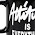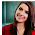# Computer System Architecture Questions and Answers 121 to 130

## Computer System Architecture

### Questions 121 to 130

121.
Of the following, what is a input device for which finger shows the cursor movement.
 (a) Mouse (b) Scanner (c) Trackball (d) Glide pad (e) Microphone.
122.
Which of the following describes the Mnemonic SPA?
 (a) Skip If (Accumulator) Positive (b) Skip If (Adder-Subtractor) Positive (c) Skip If (Associative Mapping) Positive (d) Skip If (Assembler) Positive (e) Skip If (Address Resister) Positive.
123.
Of the following, identify the memory usually written by the manufacturer.
 (a) RAM (b) ROM (c) DRAM (d) SRAM (e) Cache Memory.
124.
Consider that the program is executed from main memory until it attempts to reference a page that is still in auxiliary memory. Identify this condition.
 (a) Page Found (b) Page Fault (c) Page Hit (d) Page Miss (e) Page Replace.
125.
What is the symbol to represent a state in the state diagram?

126.
A computer has memory of 256k words of 32 bits each, how many bits are required to specify the address part?
 (a) 16 bits (b) 12 bits (c) 8 bits (d) 18 bits (e) 10 bits.
127.
V is a symbol for
 (a) OR (b) XOR (c) AND (d) NAND (e) NOR.
128.
What is represented by D7I’T in the instruction cycle?
 (a) Input-Output Instructions (b) Register–reference instructions (c) Program interrupt (d) Branch instruction (e) Memory Reference Instructions.
129.
What is described by the Mnemonic SHRA?
 (a) Arithmetic shift left (b) Logical shift right (c) Logical shift left (d) Arithmetic shift right (e) Circular shift right.
130.
CISC stands for:
 (a) Control Instruction Set Completeness (b) Complex Instruction Set Computer (c) Complex Instruction Set Conversion (d) Control Instruction Set Computer (e) Complex Instruction Set Control.

Answers

 Answer Reason 121. d Is the input device for which finger shows the cursor movement 122. a SPA stands for Skip If (Accumulator) Positive 123. b ROM is the memory usually written by the manufacturer. 124. B The program is executed from main memory until it attempts to reference a page that is still in auxiliary memory called Page Fault. 125. B is the symbol which represent a state in the state diagram 126. A A computer has memory of 256k words of 32 bits each, 18 bits are required to specify the address part. 127. A V  is a symbol for OR 128. B D7I’T  in the instruction cycle represents Register –reference instructions 129. D SHRA stands for Arithmetic shift right 130. B CISC stands for complex instruction set computer.

29  30  31  32  33  34  35  36  37  38  39  Next >>

#### 2 comments :

1.question no 102 is wrong write answer is D stand for Delay

2.Needed to compose you a very little word to thank you yet again regarding the nice suggestions you’ve contributed here.

Python Training in Bangalore